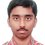Another range problem

Find the range of the function defined by f(x)=($x^{4}$ - $\sqrt{2}$x + 2)/($x^{4}$ - $\sqrt{2}$x + 1).Note by Nishant Sharma
6 years, 5 months ago

This discussion board is a place to discuss our Daily Challenges and the math and science related to those challenges. Explanations are more than just a solution — they should explain the steps and thinking strategies that you used to obtain the solution. Comments should further the discussion of math and science.

When posting on Brilliant:

• Use the emojis to react to an explanation, whether you're congratulating a job well done , or just really confused .
• Ask specific questions about the challenge or the steps in somebody's explanation. Well-posed questions can add a lot to the discussion, but posting "I don't understand!" doesn't help anyone.
• Try to contribute something new to the discussion, whether it is an extension, generalization or other idea related to the challenge.
• Stay on topic — we're all here to learn more about math and science, not to hear about your favorite get-rich-quick scheme or current world events.

MarkdownAppears as
*italics* or _italics_ italics
**bold** or __bold__ bold

- bulleted
- list

• bulleted
• list

1. numbered
2. list

1. numbered
2. list
Note: you must add a full line of space before and after lists for them to show up correctly
paragraph 1

paragraph 2

paragraph 1

paragraph 2

> This is a quote
This is a quote
# I indented these lines
# 4 spaces, and now they show
# up as a code block.

print "hello world"
# I indented these lines
# 4 spaces, and now they show
# up as a code block.

print "hello world"
MathAppears as
Remember to wrap math in $$...$$ or $...$ to ensure proper formatting.
2 \times 3 $2 \times 3$
2^{34} $2^{34}$
a_{i-1} $a_{i-1}$
\frac{2}{3} $\frac{2}{3}$
\sqrt{2} $\sqrt{2}$
\sum_{i=1}^3 $\sum_{i=1}^3$
\sin \theta $\sin \theta$
\boxed{123} $\boxed{123}$

Sort by:

I got the lower bound. But don't know how to get the upper bound. Additionally can anyone help me to find the roots of the denominator of the given function f(x)(if they exist) and if they don't then how ?

- 6 years, 5 months ago

Letting x^4 - (rt2)x + 1 = y, you get f(x) = 1 + 1/y. Some work gives you y >= 1/4, giving you a maximum of 5.

- 6 years, 5 months ago

That is what I could not get. Since the denominator function is increasing and decreasing in certain intervals, so I couldn't figure out how to obtain upper bound.

- 6 years, 5 months ago

Use f'(x) to find out intervals in which the function is increasing or decreasing. You don't need the denominator in that case.

- 6 years, 5 months ago

here nishant ; ..... i think i could help you with only the answer but not the procedure how to solve it...if you don't mind i am here posting the link from where i could find answer of this question....hope this answer(only) helps you ......

- 6 years, 5 months ago

That was fine. Understood. Did you see my recent post ? I mean roots of denominator ?

- 6 years, 5 months ago

but i could not find it....is it in the discussion forum...?...then give me the heading that you gave...

- 6 years, 5 months ago

Split the function into $1+\frac{1}{(x^4-\sqrt{2}x+1)}$

Since it is easy to see that the lower bound will be greater than 1 but never equal to one. Now for upper bound we can find the local maxima.

$f'(x)=-\frac{4x^3-\sqrt{2}}{(x^4-\sqrt{2}x+1)^2}$

Equating $f'(x)=0$

We get $x=\frac{1}{\sqrt{2}}$

We can check whether it is the local maxima or not using the second derivative test(checked it).

Now substituting for $x=\frac{1}{\sqrt{2}}$ in $f(x)$ We have $f(\frac{1}{\sqrt{2}})=5$.

So range is$(1,5]$.

- 6 years, 5 months ago

That was nicely explained. Got it.

- 6 years, 5 months ago